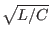Next: Uniform Distributed RC Model Up: Passive Element Lines Previous: Transmission Line Model   Contents   Index

## Uniform RC Line

General Form:
uname n1 n2 n3 modname l=len [n=lumps]
Examples:
u1 1 2 0 urcmod l=50u
urc2 1 12 2 umodl l=1mil n=6

The n1 and n2 are the two element nodes the RC line connects, while n3 is the node to which the capacitances are connected. The modname is the model name, len is the length of the RC line in meters, and lumps, if specified, is the number of lumped segments to use in modeling the RC line. If not specified, the value will be computed as

N =where N is the number of lumps, k is the proportionality factor, R and C are the total values for the length, and Fmax is the maximum frequency.

Stephen R. Whiteley 2020-07-23